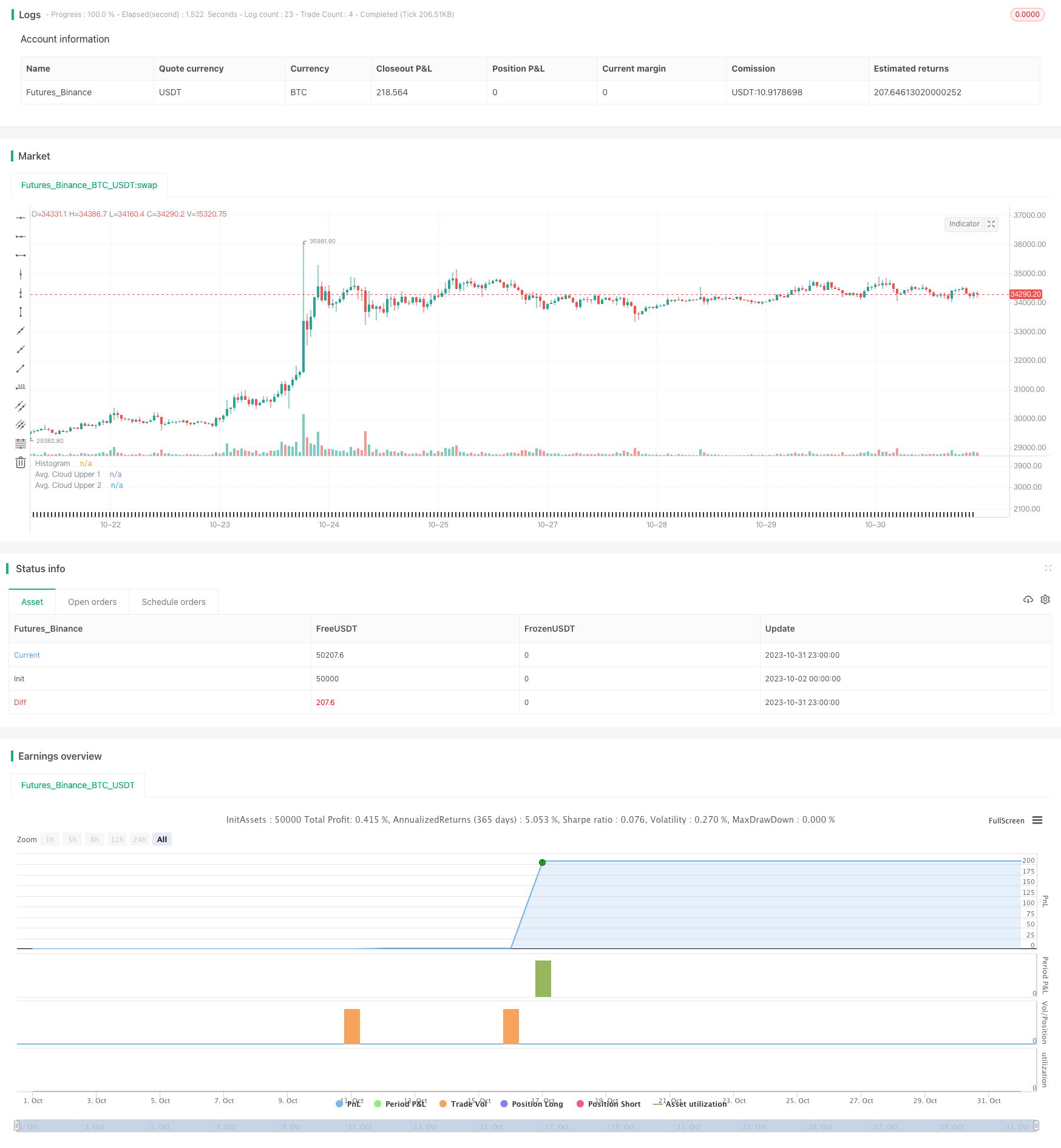# Joanne on Crypto - Dual Moving Average with MACD Scalping Strategy

Author: ChaoZhang, Date: 2023-11-02 16:09:08
Tags:## Overview

The core idea of this strategy is to combine dual moving averages and MACD indicator to determine trend direction for trend following trading. When the fast MA crosses above the slow MA, it signals an uptrend opportunity. When the fast MA crosses below the slow MA, it signals a downtrend opportunity. The MACD histogram is used to determine specific entry and exit points by going long when it crosses above 0 and going short when it crosses below 0.

## Strategy Logic

1. Calculate the fast EMA (12-day), slow EMA (26-day), and signal EMA (9-day) of MACD.

2. Calculate the MACD histogram (fast EMA - slow EMA) and MACD signal line (9-day EMA of MACD histogram).

3. Calculate the 50-day and 200-day MAs as trends.

4. MACD histogram crossing above 0 is the bullish signal and crossing below 0 is the bearish signal.

5. Fast EMA crossing above slow EMA combined with short MA crossing above long MA gives bullish signals.

6. Fast EMA crossing below slow EMA combined with short MA crossing below long MA gives bearish signals.

7. Limit number of trades after each MA crossover using Max trades after EMA cross parameter.

8. Use stop loss and take profit to exit trades.

1. Dual MAs determine overall trend to avoid counter-trend trades.

2. MACD identifies entry and exit points to capture trend shifts.

3. Combination provides good timing for entries in the direction of trend.

4. Limits number of trades after crossover to avoid chasing trends.

5. Stop loss and take profit controls risk.

6. Parameters can be optimized for better performance.

## Risks

1. Wrong trend determination leads to counter-trend loss. Widen MA difference requirement to firmly establish trend.

2. MACD signals lag price action resulting in premature or late entries. Adjust MACD parameters or add filters.

3. Improper stop loss and take profit levels lead to excessive stops or insufficient profits. Requires parameter optimization for each instrument.

4. Parameter optimization is difficult. Different parameters combinations needed for different products and timeframes. Requires extensive upfront testing.

## Enhancement Opportunities

1. Test other indicators like KD to determine trend.

2. Add other indicators to filter MACD signals, like Bollinger Bands, ATR stops.

3. Optimize stop loss and take profit for each product.

4. Utilize walkforward and random optimization to find better parameters.

5. Add mechanisms to reduce trade frequency, like MACD zone around 0.

6. Automate parameter and combination optimization across multiple products.

## Summary

This strategy combines the strengths of dual MAs for trend direction and MACD for entry timing to create a robust trend following system. Additional performance gains are possible through parameter optimization and combining indicators. Overall, it has strong risk management and profit potential to be considered for live trading. But parameter testing is still required for each product to ensure robustness.

```/*backtest
start: 2023-10-02 00:00:00
end: 2023-11-01 00:00:00
period: 1h
basePeriod: 15m
exchanges: [{"eid":"Futures_Binance","currency":"BTC_USDT"}]
*/

//@version=5
strategy(title="ComiCo - Joel on Crypto - MACD Scalping", shorttitle="ComiCo - Joel on Crypto - MACD Scalping")
// Getting inputs
slow_length1 = input(title="EMA Trend 1", defval=50)
slow_length2 = input(title="EMA Trend 2 ", defval=200)
fast_length = input(title="MACD Fast Length", defval=12)
slow_length = input(title="MACD Slow Length", defval=26)
signal_length = input.int(title="MACD Signal Smoothing",  minval = 1, maxval = 50, defval = 9)
src = input(title="MACD Source", defval=close)

i_switch = input.string(title="Tick Highlight", defval="Moving average" ,options=["Moving average","Fixed value" ])
i_switch2 = input.string(title="Tick Source", defval="Highest bar" ,options=["Highest bar","Average","Last bar"])

signal_lengthup = input.int(title="Upticks Avg. Length",  minval = 1, maxval = 5000, defval = 72)
signal_lengthdown = input.int(title="Downticks Avg. Length",  minval = 1, maxval = 5000, defval = 72)

signal_lengthMA = input.float(title="Ticks Avg. Multiplier",  minval = 0, maxval = 5000, defval = 2, step = 0.1)

sma_source = "EMA"
sma_signal = "EMA"
// Plot colors

col_grow_above = #26A69A
col_fall_above =#B2DFDB
col_grow_below = #FFCDD2
col_fall_below = #FF5252
// Calculating

fast_ma = sma_source == "SMA" ? ta.sma(src, fast_length) : ta.ema(src, fast_length)
slow_ma = sma_source == "SMA" ? ta.sma(src, slow_length) : ta.ema(src, slow_length)

time_macd=timeframe.period=="1"?"1": timeframe.period=="3"?"1": timeframe.period=="5"?"1": timeframe.period=="15"?"3":timeframe.period=="30"?"5":timeframe.period=="60"?"15":timeframe.period=="120"?"30":timeframe.period=="240"?"60":timeframe.period=="D"?"240":timeframe.period=="W"?"D":timeframe.period=="M"?"W":timeframe.period=="12M"?"M":timeframe.period

macd = fast_ma - slow_ma
macd1=request.security(syminfo.tickerid, time_macd, macd)
signal = sma_signal == "SMA" ? ta.sma(macd1, signal_length) : ta.ema(macd1, signal_length)

ema50=ta.ema(close,slow_length1)
ema200=ta.ema(close ,slow_length2)

MaxCount = input.int(title = "Max trades after EMA cross", minval = 0, maxval = 1000, defval = 3)
bull = ema50>ema200
if bull != bull

hist = request.security(syminfo.tickerid, time_macd, macd1 - signal)

f() => [hist,hist,hist,hist, hist]

a = array.from(ss5,ss4,ss3,ss2,ss1)

s3=i_switch2=="Highest bar"?(ss>0? array.max(a, 0) : array.min(a, 0)):i_switch2=="Average"?array.avg(a):i_switch2=="Last bar"?ss1:0

saa=timeframe.period == '1'? ss:s3

saa2=timeframe.period == '1'? ss:s3*signal_lengthMA

colorss=(s3>=0 ? (s3 < s3 ? col_grow_above : col_fall_above) : (s3 < s3 ? col_grow_below : col_fall_below))

saaup = saa2

saaup:=saa<0? saa2:saaup

dowww=ta.ema(saaup,signal_lengthdown)

ss22=plot(verr, title="Avg. Cloud Upper 1", color=color.new(color.white, 100))
ss33=plot(dowww, title="Avg. Cloud Lower 1", color=color.new(color.white, 100))

fill(ss22, ss33, color.new(color.white, 93), title="Avg. Cloud Background")

fixeduptick = input(title="Fixed Uptick Value", defval=30)
fixeddowntick = input(title="Fixed Downtick Value", defval=-30)
minl = i_switch=="Fixed value"? fixeduptick  :  verr
maxl = i_switch=="Fixed value"? fixeddowntick : dowww

plot(minl, title="Avg. Cloud Upper 2", color=color.new(color.white, 81))
plot(maxl, title="Avg. Cloud Lower 2", color=color.new(color.white, 81))

colors2= s3<=minl and s3>=maxl ? #2a2e39 : colorss

coro2=s3>0? ema50>ema200 ? #2a2e39 :  colors2 : ema50<ema200 ? #2a2e39: colors2
plot(saa, title="Histogram", style=plot.style_columns, color=coro2)

LimitDiff = input.float(title="Limit Price Difference",  minval = 0, maxval = 0.1, defval = 0.005, step = 0.0005)
TP = input.float(title="Take Profit",  minval = 0, maxval = 0.1, defval = 0.005, step = 0.0005)
SL = input.float(title="Stop Loss",  minval = 0, maxval = 0.1, defval = 0.004, step = 0.0005)

minEMAdiff = input.float(title = "Min EMA difference", defval = 100, step = 10)

if #2a2e39 != coro2
a22 = 0
LimitPrice = close * (1 + LimitDiff)
strategy.entry("enter short", strategy.short, limit = LimitPrice)
strategy.exit("exit short", "enter short", limit = LimitPrice * (1 - TP), stop = LimitPrice * (1 + SL))